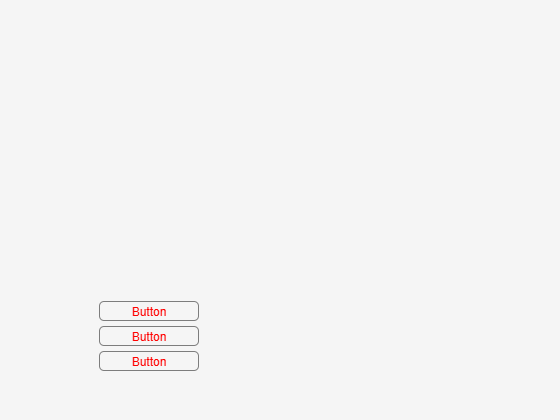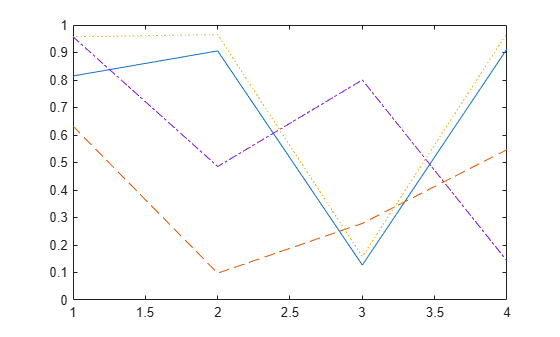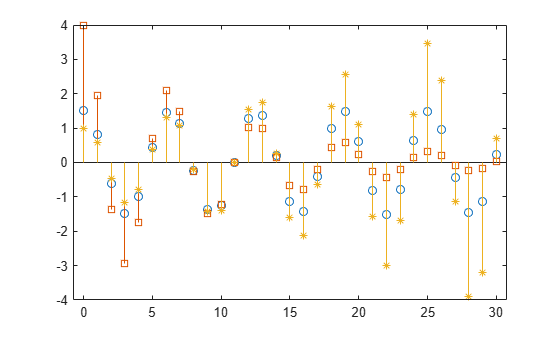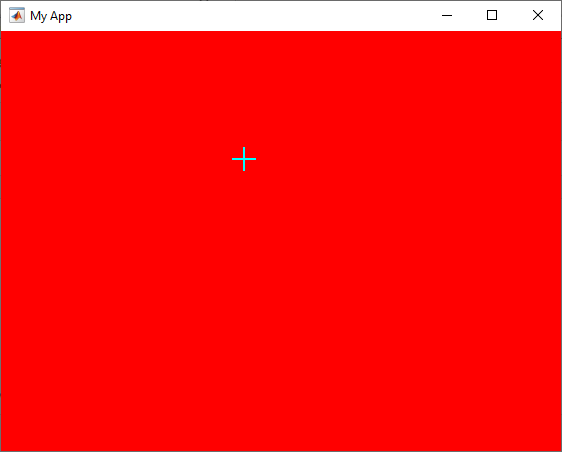# set

Set graphics object properties

## Syntax

``set(h,Name,Value)``
``set(h,defaultTypeProperty,defaultValue)``
``set(h,NameArray,ValueArray)``
``set(h,a)``
``s = set(h)``
``v = set(h,propertyName)``

## Description

example

````set(h,Name,Value)` sets properties for the specified graphics object `h` using one or more name-value arguments. If `h` is a vector of objects, then `set` sets the properties for all the objects in `h`. If `h` is empty ([ ]), `set` does nothing and does not return an error or warning.For more information about properties that you can set, see the property pages for each object, for example, Figure Properties, Axes Properties, Line Properties, and Text Properties.```
````set(h,defaultTypeProperty,defaultValue)` changes the default value of the specified property and object type for the specified graphics object `h` using one or more pairs of property names and values. `defaultTypeProperty` is the word `default` concatenated with the object type (for example, `Figure`) and the property name (for example, `Color`) in quotes. For example, `set(groot,"defaultFigureColor","red")` changes the default value of the `Color` property of `Figure` objects to `red` for the graphics root object, `groot`.```

example

````set(h,NameArray,ValueArray)` sets multiple properties for the specified graphics object `h`.If `h` is a scalar object, specify `NameArray` and `ValueArray` as 1-by-n cell arrays containing one or more property names or values, where n is the number of properties being set.If `h` is a vector of objects, to set a different property value for each object in `h`, specify `ValueArray` as an m-by-n cell array, where m is the number of elements in `h` and n is the number of property names contained in `NameArray`.```

example

````set(h,a)` sets multiple properties using `a`, which is a structure whose field names are the object property names and whose field values are the corresponding property values. If `a` is empty, `set` does nothing and does not return an error or warning.```

example

````s = set(h)` returns the user-settable properties and possible values for the specified graphics object `h`. `h` must be a single object. This syntax does not change the properties of `h`.If `s` is not specified, `set` displays the user-settable properties and possible values in the Command Window.```
````v = set(h,propertyName)` returns the possible values for the specified property. If the possible values are character vectors or strings, `set` returns a cell array containing the values. For other properties that do not have a fixed set of values, `set` returns an empty cell array. `h` must be a single object. This syntax does not change the properties of `h`.If `v` is not specified, `set` displays the possible values in the Command Window.```

## Examples

collapse all

Create a line plot and return the `Line` object as `p`. Set the `Color` property of the line to `"red"`.

```p = plot(1:10); set(p,"Color","red")```Create a figure with three buttons and put them into the array `btns`. Set the `FontColor` property of all the buttons to `"red"`.

```fig = uifigure; btn1 = uibutton(fig,"Position",[100 100 100 20]); btn2 = uibutton(fig,"Position",[100 75 100 20]); btn3 = uibutton(fig,"Position",[100 50 100 20]); btns = [btn1 btn2 btn3]; set(btns,"FontColor","red")```Create a plot with four lines using random data, and return the four `Line` objects as `p`. Set the `LineStyle` property of each of the four `Line` objects to a different value. Transpose the list of `LineStyle` values from a row vector to a column vector to match the shape of `p`.

```p = plot(rand(4)); NameArray = {'LineStyle'}; ValueArray = transpose({'-','--',':','-.'}); set(p,NameArray,ValueArray)```Plot a discrete data sequence and return the three `Stem` objects as `st`. Set the `Marker` and `Tag` properties of the three different `Stem` objects to different values. Each row of the value cell array corresponds to an object in `st` and contains two values, one for the `Marker` property and one for the `Tag` property.

```x = 0:30; y = [1.5*cos(x); 4*exp(-.1*x).*cos(x); exp(.05*x).*cos(x)]'; st = stem(x,y); NameArray = {'Marker','Tag'}; ValueArray = {'o','Decaying Exponential'; ... 'square','Growing Exponential'; ... '*','Steady State'}; set(st,NameArray,ValueArray)```Create a figure and return the user-settable properties and possible values for the figure.

```fig = uifigure; s = set(fig);```

Display the possible values for the `Pointer` property.

`s.Pointer`
```ans = 17x1 cell {'arrow' } {'ibeam' } {'crosshair'} {'watch' } {'topl' } {'topr' } {'botl' } {'botr' } {'circle' } {'cross' } {'fleur' } {'custom' } {'left' } {'top' } {'right' } {'bottom' } {'hand' } ```

Create a structure with `Name`, `Color`, and `Pointer` fields, and use the structure to set those properties for the figure.

```a.Name = "My App"; a.Color = "red"; a.Pointer = "crosshair"; set(fig,a)```## Input Arguments

collapse all

Graphics objects, specified as a single object or a vector of objects.

Note

Do not use the `set` function on Java® objects as it will cause a memory leak. For more information, see Access Public and Private Data.

Property and value pairs, specified as `Name1=Value1,...,NameN=ValueN`, where `Name` is the property name and `Value` is the corresponding value.

Each type of object supports a different set of properties. For a full list of properties and descriptions for each type, see the associated object property page (for example, Figure Properties, Axes Properties, Line Properties, and Text Properties).

To set property values, specify the name followed by an equal sign (`=`) and the corresponding value. For example, `set(h,Color="red")`.

Before R2021a, use commas to separate each name and value, and enclose `Name` in quotes. For example, `set(h,"Color","red")`.

To set a property to its default value, specify the property value as the word `default` in quotes. For example, `set(h,"Color","default")`.

Default property and value pairs, specified as `defaultTypeProperty1=defaultValue1,...,defaultTypePropertyN=defaultValueN`, where `defaultTypeProperty` is the word `default` concatenated with the object type (for example, `Figure`) and the property name (for example, `Color`), and `defaultValue` is the corresponding default value.

To change default property values, specify `defaultTypeProperty` followed by an equal sign (`=`) and the corresponding default value. For example, `set(h,defaultFigureColor="red")`.

Before R2021a, use commas to separate each name and value, and enclose `Name` in quotes. For example, `set(h,"defaultFigureColor","red")`.

To remove the default value for a property, specify the property value as the word `remove` in quotes. For example, `set(h,"defaultFigureColor","remove")`.

Property names, specified as a cell array containing one or more property names.

Property values, specified as an m-by-n cell array, where m is the number of elements in `h` and n is the number of property names contained in `NameArray`.

Object property names and values, specified as a structure whose field names are the object property names and whose field values are the corresponding property values.

Property name, specified as a string scalar or character vector.

## Output Arguments

collapse all

Property names and their values, returned as a structure. In the structure, the field names are the object property names, and the field values are the possible values of the corresponding properties.

Possible property values, returned as a cell array. If the possible values are character vectors or strings, `set` returns a cell array containing the values. For other properties that do not have a fixed set of values, `set` returns an empty cell array.

## Tips

• When setting the `FontSize` and `FontUnits` properties in the same `set` statement, specify the `FontUnits` property first. This order allows the `set` function to interpret the specified `FontSize` as intended. Similarly, when setting figure and axes units, set the `Units` property before setting additional properties whose values are calculated using the specified units. For example, this command sets the `Units` property before setting the `Position` property, whose measurements are in units specified by the `Units` property.

```f = figure; set(f,"Units","characters","Position",[30 30 120 35]);```

## Version History

Introduced before R2006a